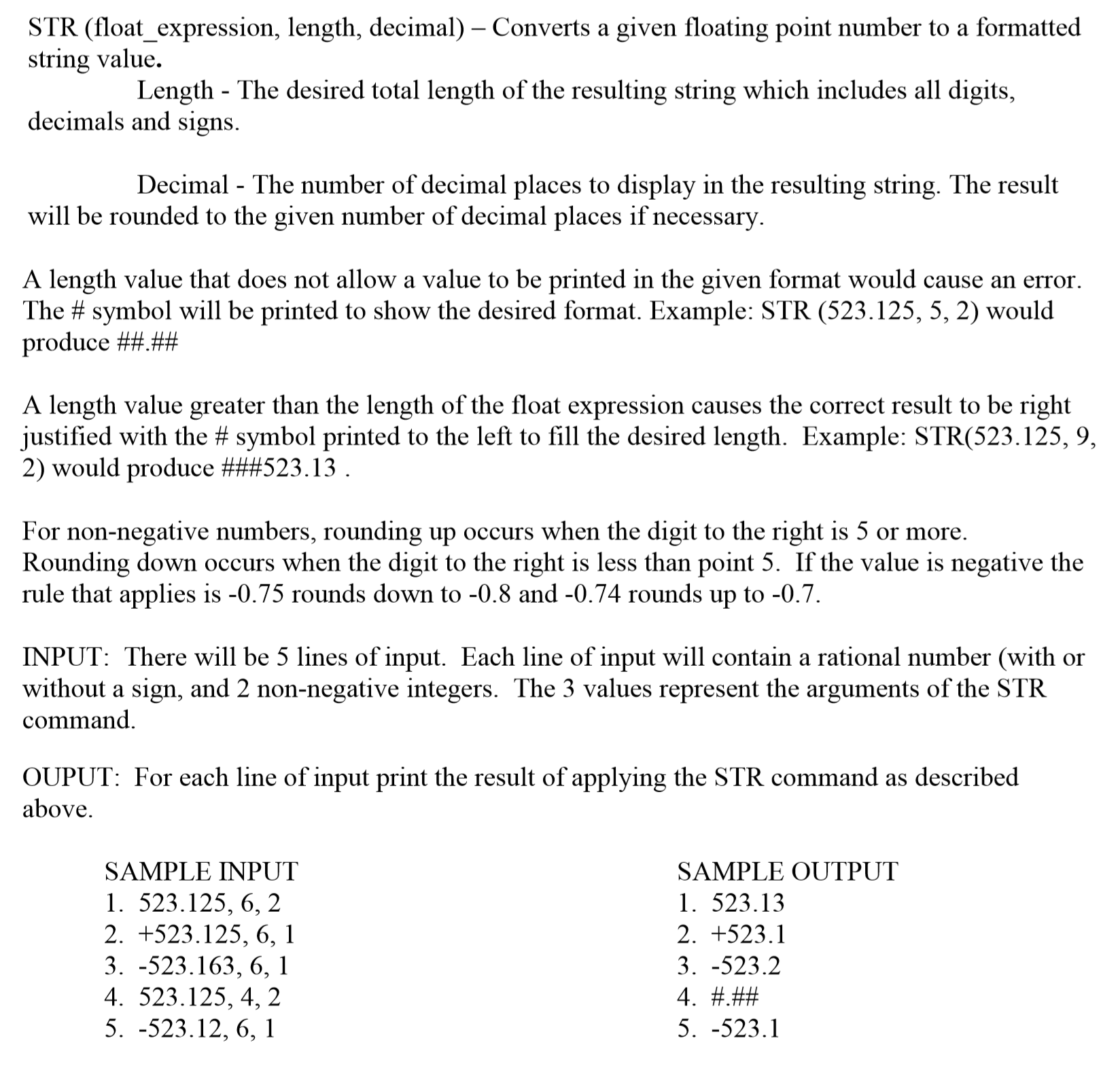# ACSL STRING

## Question## My thought

Nothing… The problem is pretty easy, however it troubled me for a quite long time. Just follow the instruction of the problem and simulate the whole process by coding.

## Points to note

• The conversion between String and int
• How to handle carry
• Pay attention to the additional sign
• Sometimes we can use char to simplify the process

# 济南Day3 坐标型动态规划及背包

## 花店橱窗布置

### 思路

• f[i][j]f[i][j]表示前i个花瓶前j个花束的最大美学价值
• f[i][j]=max(f[i−1][k],f[i][j])f[i][j]=max(f[i−1][k],f[i][j])

• 整张表都是向右下方走的，向下走加上数值，向右走无影响
• 如果向下走代表花束放入花瓶，向右走无影响代表不放入

## 矩阵取数

### 思路

• 因为每次取头和尾，所以一定是一个连续的区间
• f[i][j]f[i][j]表示从i取到j的最大得分
• $f[i][j]=max(f[i][j-1]+a[j]2^(i-1+n-j+1+1) \ , \ f[i-1][j]+a[i]2^(i-1+n-j+1+1))$
• 指数是轮数，当前数前面有多少个数被取走，就有多少轮，注意一下1的问题

## 传纸条

### 思路

• f[i][j][k][l]f[i][j][k][l]表示现在去的到了(i,j),回来的到了(k,l)

• 一共有四种情况，上上，左左，上左，左上

• 保证两条路径不相交if(j1==j2 && i1==i2) continue

• f[i][j][k][l]=max(f[i−1][j][k−1])f[i][j][k][l]=max(f[i−1][j][k−1])

• 步数为i+j−1i+j−1

• 判断不合法的状态

• 当m,n<100m,n<100时，四维数组开不下了，因为i1+j1=i2+j2i1+j1=i2+j2的时候才是合法的，并且三个数都确定时，我们可以直接算出j2j2,直接变成了三维

## 免费馅饼

### 思路

• f[i][j]f[i][j]表示第i秒到达第j个格子的最大得分
• 该状态是从哪里转移过来的呢？ 他可以从5个地方转移过来（没有到边界的时候）
• 运动具有相对性，我们可以把该问题类比成数字三角形，相当于馅饼不动，人每次向上移动一个单位
• f[i][j]=ff[i][j]=f

## 三角蛋糕

### 思路

• 像数字三角形一样压缩成正三角形
• f[i][j]=min(f[i+1][j],f[i+1][j+1])+1f[i][j]=min(f[i+1][j],f[i+1][j+1])+1

## 金明的预算方案

### 思路：分组背包

• f[i][j]f[i][j]表示前i组物品重量不超过j的最大价值
• 一共5种转移方式
• f[i][j]=max(f[i−1][j],f[i−1][j−w[i][k]]+v[i][k]),k=1,2,3,4,5f[i][j]=max(f[i−1][j],f[i−1][j−w[i][k]]+v[i][k]),k=1,2,3,4,5,五种情况已经重新配置的前提下

## 01/完全背包混合

• 根据物品的类型选择状态转移方程

## 二维限制的背包

• 把数组扩展成三维
• f[i][j][k]=max(f[i−1][j][k],f[i−1][j−w[i]][k−v[i]]+c[i])f[i][j][k]=max(f[i−1][j][k],f[i−1][j−w[i]][k−v[i]]+c[i])

## 面包

### 思路

• 如果没有面包被压扁，就是一个完全背包问题
• 如果有大面包，肯定要放到最上面，使得压缩高度尽可能大
• 枚举最上面是哪一个大面包，然后其余所有面包的高度都变为原来的五分之四，就转化成了一半的完全背包问题

# 树形DP

## 二叉苹果树

• $dp[u][j表示节点u留下j条边的最大价值，每一次决策只有三种情况：剪左子树，剪右子树，两个都不剪 • 剪左边：dp[u][j]=dp[rson][j−1]+v[rson]dp[u][j]=dp[rson][j−1]+v[rson]，同理，剪右边：dp[u][j]=dp[lson][j−1]+v[lson]dp[u][j]=dp[lson][j−1]+v[lson] • 两边都不剪：dp[u][j]=dp[lson][j]+dp[rson][k−j−2]dp[u][j]=dp[lson][j]+dp[rson][k−j−2] ## 代码：记忆化搜索 ## 先修课 ### 题目 课的先修关系形成一棵树的结构，每门课有一门或者没有先修课。选M门课，能获得的最大学分是多少？ ### 思路 • dp[u][j]dp[u][j]以u为根的子树选j门课 • 把j-1门课分配给子树，就是一个背包？ • 然后我们用f[i][j]f[i][j]表示当前树中，前i棵子树选择j门课的最大学分。这样就能够通过f算出dp[i][j]dp[i][j],相当于树上DP套背包。对于每一个状态dp[i][j]dp[i][j]都需要用f算出学分分配的最佳方案 ## K-set ### 题目 在一棵树中选出最多的点，使得这些点中每个点最多与其他点连了k条边 ### 思路 • dp[u][0/1][0/1]dp[u][0/1][0/1]表示以u为父亲，取/不取父亲,取/不取自己的最大值 • dp[u]dp[u]：自己和父亲都不取，u的儿子随便取。$f[u]=size∑i=1max( dp(vi,0,0) , dp(vi,0,1) )f[u]=∑i=1sizemax( dp(vi,0,0) , dp(vi,0,1) )\$

• dp[u]dp[u]：父亲要取，u自己不取，u的儿子同样随便取。f[u]=f[u]f[u]=f[u]

• dp[u]dp[u]

：父亲要取，自己也要取，儿子取k-1条边。

• 这时我们就要考虑取哪几条边，也就是哪几个点。
• 对于任意一个u的儿子vivi,如果取这个点，那么它的贡献就是dp(vi,1,1)dp(vi,1,1)，如果不取这个点，那么它的贡献就是dp(vi,1,0)dp(vi,1,0)
• 因此这个点取或者不取，带来的贡献就是两种情况的差，所以我们只要按两种情况的差从大到小排序就好了。最后选取差值最大的k-1个点就OK了（前提是这k-1个点带来的贡献都是正的，如果有负贡献那么就取不满k-1个点）
• dp[u]dp[u]：父亲不取，自己要取，儿子取k条边

# 题意

44的棋盘，一共有三种属性：白棋，黑棋，空格（有且仅有两个），每一次可以移动一颗棋子，*黑白棋交替进行，只能移到空格的地方。求达成四子连棋局面（横竖斜都算**）所需的最小步数

# 分析

• 广搜，和八数码问题差不多，但是更繁琐了。
• 黑白棋交替进行，那么我们需要在搜索的时候除了当前地图和步数还需要保存当前该哪一方行棋
• 广搜要搜两遍，分别是黑棋先走或白棋先走
• 每一次需要考虑两个空格，所以从两个当前点搜状态

# 遇到的坑

• 很多都是重复的代码，只要细心就好了，但有一个最坑的……\

# STL

C++ STL 之所以得到广泛的赞誉，也被很多人使用，不只是提供了像vector, string, list等方便的容器，更重要的是STL封装了许多复杂的数据结构算法和大量常用数据结构操作。vector封装数组，list封装了链表，map和set封装了二叉树等，在封装这些数据结构的时候，STL按照程序员的使用习惯，以成员函数方式提供的常用操作，如：插入、排序、删除、查找等。让用户在STL使用过程中，并不会感到陌生。

# SET

## set的各成员函数列表

1. begin()–返回指向第一个元素的迭代器
2. clear()–清除所有元素
3. count()–返回某个值元素的个数
4. empty()–如果集合为空，返回true
5. end()–返回指向最后一个元素的迭代器
6. equal_range()–返回集合中与给定值相等的上下限的两个迭代器
7. erase()–删除集合中的元素
8. find()–返回一个指向被查找到元素的迭代器
9. get_allocator()–返回集合的分配器
10. insert()–在集合中插入元素
11. lower_bound()–返回指向大于（或等于）某值的第一个元素的迭代器
12. key_comp()–返回一个用于元素间值比较的函数
13. max_size()–返回集合能容纳的元素的最大限值
14. rbegin()–返回指向集合中最后一个元素的反向迭代器
15. rend()–返回指向集合中第一个元素的反向迭代器
16. size()–集合中元素的数目
17. swap()–交换两个集合变量
18. upper_bound()–返回大于某个值元素的迭代器
19. value_comp()–返回一个用于比较元素间的值的函数

# P1284三角形牧场

## 分析

• 首先看数据范围，边长最大为40*40/3，并且因为要使用所有的木棍，所以只要两条边确定就可以知道第三条边的确定长度。因此我们可以设状态f[i][j]表示三角形的两条边分别是i，j的情况是否成立

• f[i][j]=f[i−a[k]][j] || f[i][j−a[k]] || f[i][j]f[i][j]=f[i−a[k]][j] || f[i][j−a[k]] || f[i][j]，相当于01背包，就不解释了……

• 定义初始状态f=1f=1

• 三条边都知道了，如何求面积呢？这里我们需要用到海伦公式

S=√p(p−a)(p−b)(p−c)S=p(p−a)(p−b)(p−c)，p=12(a+b+c)p=12(a+b+c)

# P1929 迷之阶梯

## 题意

1. 如果下一步阶梯的高度只比当前阶梯高 1，则可以直接登上。
2. 除了第一步阶梯外，都可以从当前阶梯退到前一步阶梯。
3. 当你连续退下 k 后，你可以一次跳上不超过当前阶梯高度 2^{k}2k的阶梯。比如说你现 在位于第 j 步阶梯，并且是从第 j+k 步阶梯退下来的，那么你可以跳到高度不超过当前阶 梯高度+2^{k}2k的任何一步阶梯。跳跃这一次只算一次移动。

## 分析

• 定义状态为f[i]表示到第i个阶梯的最小步数
• 由2可以直接得出if(h[i]==h[i-1]+1) f[i]=f[i-1]+1

## 遇到的坑

• if(f[n]>=0x3f3f3f3f) f[n]=-1;在状态转移的过程中有可能比初始值更大，所以是大于等于